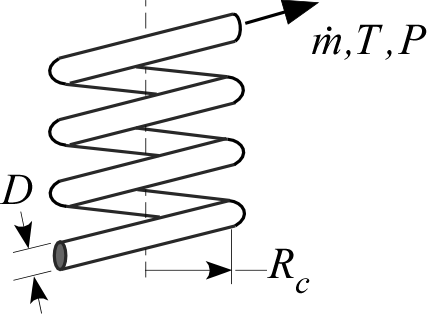Contents - Index

HelicalPipeFlow_ND_localProcedure HelicalPipeFlow_ND_local(Re, Pr, x\D, RelRough,D\R_c: Nusselt_T, Nusselt_H, f) returns the local Nusselt number assuming constant temperature wall and constant heat flux for a given flow condition in a helically wound circular tube characterized by Reynolds and Prandtl numbers, length/diameter, relative roughness, and diameter to coil radius ratio. The Procedure also returns the local friction factor for these conditions. The procedure can be used for developing or fully-developed flow in the laminar, transitional or turbulent flow regimes.

Inputs:

Re - Reynolds number based on properties evaluated at the bulk average temperature

Pr - Prandtl number of the fluid evaluated at the bulk average temperature

x\D - ratio of axial position to diameter.

RelRough - the ratio of the dispersions on the wall of the tube to the tube diameter (must be between 0 and 0.05)

D\R_c - the ratio of the tube inner diameter to the radius of the coil

Outputs:

Nusselt_T - local Nusselt number assuming a constant wall temperature

Nusselt_H - local Nusselt number assuming a constant wall heat flux

f - local friction factor [-]

Notes

This procedure is called by HelicalPipeFlow_local. It calls PipeFlow procedures and then modifies these straight tube results in order to account for the curvature of the coil using correlations discussed in the Heat Exchanger Design Handbook (2008) and Barron (1999)

Two Nusselt numbers are returned. Nusselt_T corresponds to a constant temperature wall and Nusselt_H corresponds to a constant heat flux. These two values will be the same for turbulent flow. For laminar flow, they provide upper and lower bounds on the Nusselt number as explained in Section 5.2.4 of  Nellis and Klein.

Example

Re=10000

Pr=2

x\D=100

relRough=0

D\R_c=0.2

Call HelicalPipeFlow_ND_local(Re, Pr, x\D, relRough,D\R_c: Nusselt_T,Nusselt_H, f)

{Solution:

f=0.186 [-]

Nusselt_H=72.71

Nusselt_T=72.71 [-]}